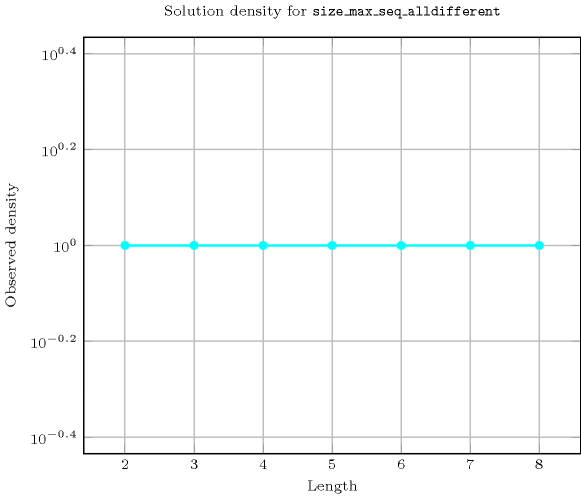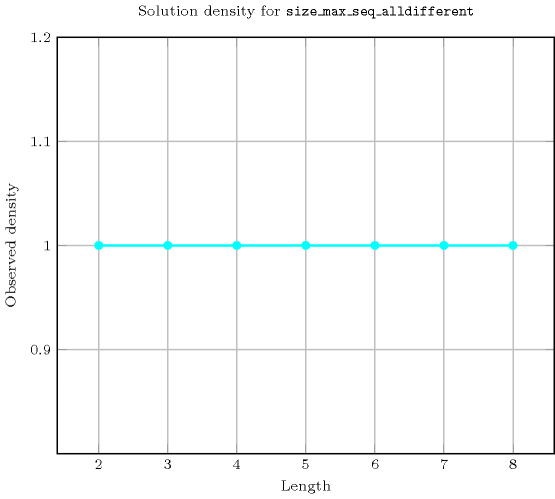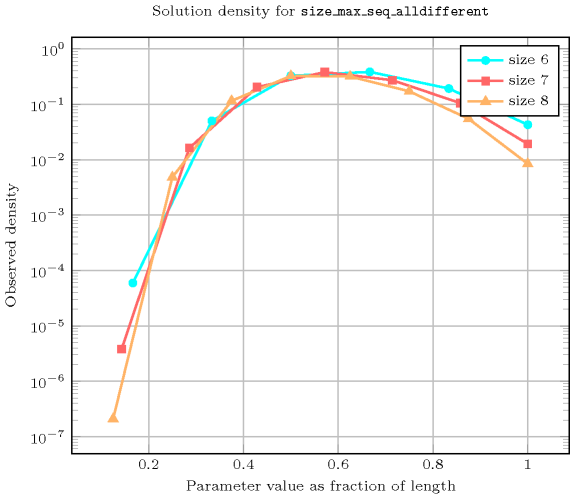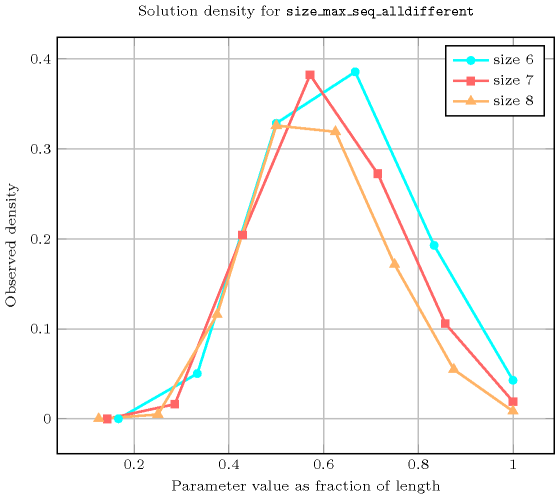## 5.347. size_max_seq_alldifferent

Origin

N. Beldiceanu

Constraint

$\mathrm{𝚜𝚒𝚣𝚎}_\mathrm{𝚖𝚊𝚡}_\mathrm{𝚜𝚎𝚚}_\mathrm{𝚊𝚕𝚕𝚍𝚒𝚏𝚏𝚎𝚛𝚎𝚗𝚝}\left(\mathrm{𝚂𝙸𝚉𝙴},\mathrm{𝚅𝙰𝚁𝙸𝙰𝙱𝙻𝙴𝚂}\right)$

Synonyms

$\mathrm{𝚜𝚒𝚣𝚎}_\mathrm{𝚖𝚊𝚡𝚒𝚖𝚊𝚕}_\mathrm{𝚜𝚎𝚚𝚞𝚎𝚗𝚌𝚎}_\mathrm{𝚊𝚕𝚕𝚍𝚒𝚏𝚏}$, $\mathrm{𝚜𝚒𝚣𝚎}_\mathrm{𝚖𝚊𝚡𝚒𝚖𝚊𝚕}_\mathrm{𝚜𝚎𝚚𝚞𝚎𝚗𝚌𝚎}_\mathrm{𝚊𝚕𝚕𝚍𝚒𝚜𝚝𝚒𝚗𝚌𝚝}$, $\mathrm{𝚜𝚒𝚣𝚎}_\mathrm{𝚖𝚊𝚡𝚒𝚖𝚊𝚕}_\mathrm{𝚜𝚎𝚚𝚞𝚎𝚗𝚌𝚎}_\mathrm{𝚊𝚕𝚕𝚍𝚒𝚏𝚏𝚎𝚛𝚎𝚗𝚝}$.

Arguments
 $\mathrm{𝚂𝙸𝚉𝙴}$ $\mathrm{𝚍𝚟𝚊𝚛}$ $\mathrm{𝚅𝙰𝚁𝙸𝙰𝙱𝙻𝙴𝚂}$ $\mathrm{𝚌𝚘𝚕𝚕𝚎𝚌𝚝𝚒𝚘𝚗}\left(\mathrm{𝚟𝚊𝚛}-\mathrm{𝚍𝚟𝚊𝚛}\right)$
Restrictions
 $\mathrm{𝚂𝙸𝚉𝙴}\ge 0$ $\mathrm{𝚂𝙸𝚉𝙴}\le |\mathrm{𝚅𝙰𝚁𝙸𝙰𝙱𝙻𝙴𝚂}|$ $\mathrm{𝚛𝚎𝚚𝚞𝚒𝚛𝚎𝚍}$$\left(\mathrm{𝚅𝙰𝚁𝙸𝙰𝙱𝙻𝙴𝚂},\mathrm{𝚟𝚊𝚛}\right)$
Purpose

$\mathrm{𝚂𝙸𝚉𝙴}$ is the size of the maximal sequence (among all possible sequences of consecutive variables of the collection $\mathrm{𝚅𝙰𝚁𝙸𝙰𝙱𝙻𝙴𝚂}$) for which the $\mathrm{𝚊𝚕𝚕𝚍𝚒𝚏𝚏𝚎𝚛𝚎𝚗𝚝}$ constraint holds.

Example
 $\left(4,〈2,2,4,5,2,7,4〉\right)$ $\left(1,〈2,2,2,2,2,2,2〉\right)$ $\left(2,〈2,2,4,4,4,7,4〉\right)$ $\left(7,〈2,0,4,6,5,7,3〉\right)$

The first $\mathrm{𝚜𝚒𝚣𝚎}_\mathrm{𝚖𝚊𝚡}_\mathrm{𝚜𝚎𝚚}_\mathrm{𝚊𝚕𝚕𝚍𝚒𝚏𝚏𝚎𝚛𝚎𝚗𝚝}$ constraint holds since the constraint $\mathrm{𝚊𝚕𝚕𝚍𝚒𝚏𝚏𝚎𝚛𝚎𝚗𝚝}$$\left(〈\mathrm{𝚟𝚊𝚛}-4,\mathrm{𝚟𝚊𝚛}-5,\mathrm{𝚟𝚊𝚛}-2,\mathrm{𝚟𝚊𝚛}-7〉\right)$ holds and since the following three constraints do not hold:

Typical
 $\mathrm{𝚂𝙸𝚉𝙴}>2$ $\mathrm{𝚂𝙸𝚉𝙴}<|\mathrm{𝚅𝙰𝚁𝙸𝙰𝙱𝙻𝙴𝚂}|$ $\mathrm{𝚛𝚊𝚗𝚐𝚎}$$\left(\mathrm{𝚅𝙰𝚁𝙸𝙰𝙱𝙻𝙴𝚂}.\mathrm{𝚟𝚊𝚛}\right)>1$
Symmetry

One and the same constant can be added to the $\mathrm{𝚟𝚊𝚛}$ attribute of all items of $\mathrm{𝚅𝙰𝚁𝙸𝙰𝙱𝙻𝙴𝚂}$.

Arg. properties

Functional dependency: $\mathrm{𝚂𝙸𝚉𝙴}$ determined by $\mathrm{𝚅𝙰𝚁𝙸𝙰𝙱𝙻𝙴𝚂}$.

Counting
 Length ($n$) 2 3 4 5 6 7 8 Solutions 9 64 625 7776 117649 2097152 43046721

Number of solutions for $\mathrm{𝚜𝚒𝚣𝚎}_\mathrm{𝚖𝚊𝚡}_\mathrm{𝚜𝚎𝚚}_\mathrm{𝚊𝚕𝚕𝚍𝚒𝚏𝚏𝚎𝚛𝚎𝚗𝚝}$: domains $0..n$Length ($n$)2345678
Total9646257776117649209715243046721
 Parameter value

13456789
26362001050592234104208224
3-243003480386404284004981032
4--12025204536080136014028336
5---7202268057120013728960
6----50402217607378560
7-----403202358720
8------362880

Solution count for $\mathrm{𝚜𝚒𝚣𝚎}_\mathrm{𝚖𝚊𝚡}_\mathrm{𝚜𝚎𝚚}_\mathrm{𝚊𝚕𝚕𝚍𝚒𝚏𝚏𝚎𝚛𝚎𝚗𝚝}$: domains $0..n$Keywords
Arc input(s)

$\mathrm{𝚅𝙰𝚁𝙸𝙰𝙱𝙻𝙴𝚂}$

Arc generator
$\mathrm{𝑃𝐴𝑇𝐻}_𝑁$$↦\mathrm{𝚌𝚘𝚕𝚕𝚎𝚌𝚝𝚒𝚘𝚗}$

Arc arity
$*$
Arc constraint(s)
$\mathrm{𝚊𝚕𝚕𝚍𝚒𝚏𝚏𝚎𝚛𝚎𝚗𝚝}$$\left(\mathrm{𝚌𝚘𝚕𝚕𝚎𝚌𝚝𝚒𝚘𝚗}\right)$
Graph property(ies)
$\mathrm{𝐍𝐀𝐑𝐂}$$=\mathrm{𝚂𝙸𝚉𝙴}$

Graph model

Note that this is an example of global constraint where the arc constraints do not have the same arity. However they correspond to the same type of constraint.